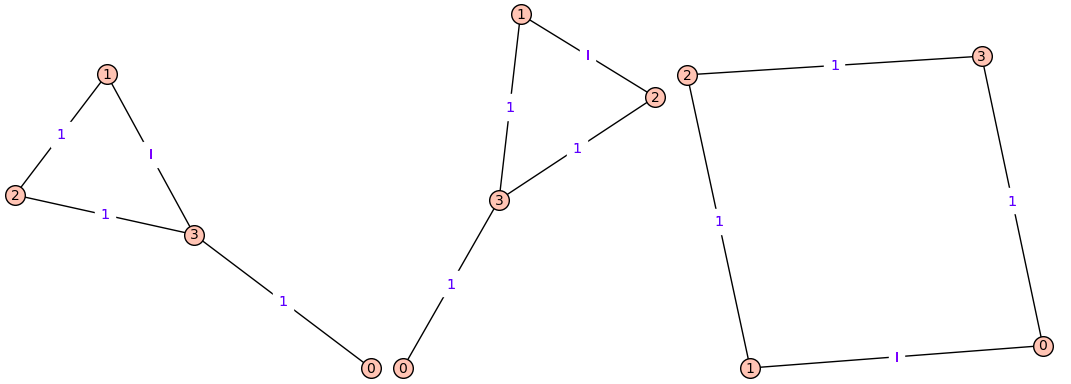# Revision history [back]

The problem can be approached with the following steps:

1. Use nauty to generate all unicyclic graphs on $n$ vertices, like in my earlier answer. In each such graph:
1. assign weight 1 to all edges
2. find its unique cycle and iteratively assign weight $i$ to each of its edges
3. use canonical labeling to eliminate duplicates

Here is a sample code:

def get_graphs(n):
S = set()
# iterate over all unicyclic graphs with n vertices
for G in graphs.nauty_geng(options=f'-c {n} {n}:{n}'):
# assign weight 1 to all edges of G
for e in G.edges(labels=False):
G.set_edge_label(e, e, 1)
# set of edges participating in cycles
E = set.union( *(set(c) for c in G.cycle_basis(output='edge')) )
for e in E:
# temporarily set weight of e to I
G.set_edge_label(e, e, I)
# restore weight of e to 1
G.set_edge_label(e, e, 1)
return S


For example,

for H in get_graphs(4): H.show(edge_labels=True)


produces 3 graphs:The problem can be approached with the following steps:

1. Use nauty to generate all unicyclic graphs on $n$ vertices, like in my earlier answer. In each such graph:
1. assign weight 1 to all edges
2. find its unique cycle and iteratively assign weight $i$ to each of its edges
3. use canonical labeling to eliminate duplicates

Here is a sample code:

def get_graphs(n):
S = set()
# iterate over all unicyclic graphs with n vertices
for G in graphs.nauty_geng(options=f'-c {n} {n}:{n}'):
# assign weight 1 to all edges of G
for e in G.edges(labels=False):
G.set_edge_label(e, e, 1)
# set of edges participating in cycles
E = set.union( *(set(c) for c in G.cycle_basis(output='edge')) )
for e in E:
# temporarily set weight of e to I
G.set_edge_label(e, e, I)
# add canonical labeling of G to set S
# restore weight of e to 1
G.set_edge_label(e, e, 1)
return S


For example,

for H in get_graphs(4): H.show(edge_labels=True)


produces 3 graphs:The problem can be approached with the following steps:

1. Use nauty to generate all connected unicyclic graphs on $n$ vertices, like in my earlier answer. In each such graph:
1. assign weight 1 to all edges
2. find its unique cycle and iteratively assign weight $i$ to each of its edges
3. use canonical labeling to eliminate duplicates

Here is a sample code:

def get_graphs(n):
S = set()
# iterate over all connected unicyclic graphs with n vertices
for G in graphs.nauty_geng(options=f'-c {n} {n}:{n}'):
# assign weight 1 to all edges of G
for e in G.edges(labels=False):
G.set_edge_label(e, e, 1)
# set of edges participating in cycles
E = set.union( *(set(c) for c in G.cycle_basis(output='edge')) )
for e in E:
# temporarily set weight of e to I
G.set_edge_label(e, e, I)
# add canonical labeling of G to set S
# restore weight of e to 1
G.set_edge_label(e, e, 1)
return S


For example,

for H in get_graphs(4): H.show(edge_labels=True)


produces 3 graphs:The problem can be approached with the following steps:

1. Use nauty to generate all connected unicyclic graphs on $n$ vertices, like in my earlier answer. In each such graph:
1. assign weight 1 to all edges
2. find its unique cycle and iteratively assign weight $i$ to each of its edges
3. use canonical labeling to eliminate duplicates

Here is a sample code:

def get_graphs(n):
S = set()
# iterate over all connected unicyclic graphs with n vertices
for G in graphs.nauty_geng(options=f'-c {n} {n}:{n}'):
# assign weight 1 to all edges of G
for e in G.edges(labels=False):
G.set_edge_label(e, e, 1)
# set of edges participating in cycles
E = set.union( *(set(c) for c in G.cycle_basis(output='edge')) )
for e in E:
# temporarily set weight of e to I
G.set_edge_label(e, e, I)
# add canonical labeling of G to set S
# restore weight of e to 1
G.set_edge_label(e, e, 1)
return S


For example,

for H in get_graphs(4): H.show(edge_labels=True)


produces 3 graphs:ADDED. This is how we get matrices requested in the comments (for $n=6$ as an example):

for H in get_graphs(6):
p = [(i,j) for i in range(A.nrows()) for j in range(i) if A[i,j]==I]
A[p] = -I
f = A.characteristic_polynomial()
h = f.reverse().subs({f.variables():-f.variables()})
if f==h:
print(A,end='\n\n')


It prints the following 3 matrices:

[ 0  0  0  1  0  0]
[ 0  0  0  0  0  1]
[ 0  0  0  0  1  I]
[ 1  0  0  0  0  1]
[ 0  0  1  0  0  1]
[ 0  1 -I  1  1  0]

[ 0  0  0  0  1  0]
[ 0  0  0  1  0  0]
[ 0  0  0  0  0  1]
[ 0  1  0  0  I  1]
[ 1  0  0 -I  0  1]
[ 0  0  1  1  1  0]

[ 0  0  0  0  0  1]
[ 0  0  0  0  1  0]
[ 0  0  0  I  0  1]
[ 0  0 -I  0  0  1]
[ 0  1  0  0  0  1]
[ 1  0  1  1  1  0]